### A.14 The Klein-Gor­don equa­tion

The Schrö­din­ger equa­tion for the quan­tum wave func­tion is based on the non­rel­a­tivis­tic ex­pres­sion for the en­ergy of a par­ti­cle. This ad­den­dum looks at the sim­plest rel­a­tivis­tic equa­tion for wave func­tions, called the Klein-Gor­don equa­tion. The dis­cus­sion will largely be re­stricted to a spin­less par­ti­cle in empty space, where there is no po­ten­tial en­ergy. How­ever, the Klein-Gor­don equa­tion is the first step to more com­plex rel­a­tivis­tic equa­tions.

Re­call first how the Schrö­din­ger equa­tion arose. If there is no po­ten­tial en­ergy, clas­si­cal physics says that the en­ergy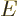is just the ki­netic en­ergy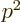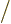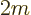of the par­ti­cle. Here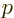is the lin­ear mo­men­tum of the par­ti­cle and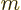its mass. Quan­tum me­chan­ics re­places the en­ergyby the op­er­a­tor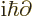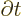and the mo­men­tum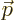by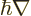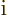, where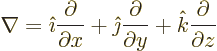Then it ap­plies the re­sult­ing op­er­a­tors on the wave func­tion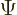. That then pro­duces the Schrö­din­ger equa­tion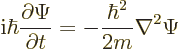So­lu­tions with a def­i­nite valuefor the en­ergy take the form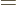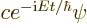. Sub­sti­tut­ing that into the Schrö­din­ger equa­tion and re­ar­rang­ing pro­duces the so-called Hamil­ton­ian eigen­value prob­lem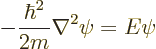Here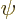is called the en­ergy eigen­func­tion.

Ac­cord­ing to clas­si­cal rel­a­tiv­ity how­ever, the en­ergyof a par­ti­cle in empty space is not just ki­netic en­ergy, but also rest mass en­ergy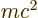, where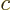is the speed of light. In par­tic­u­lar, chap­ter 1.1.2 (1.2),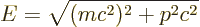The mo­men­tum can be iden­ti­fied with the same op­er­a­tor as be­fore. But square roots of op­er­a­tors are very ugly. So the smart thing to do is to square both sides above. Mak­ing the same sub­sti­tu­tions as for the Schrö­din­ger equa­tion and clean­ing up then gives the “Klein-Gor­don equa­tion”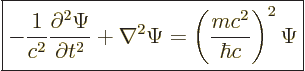(A.43)

So­lu­tionswith def­i­nite en­ergysat­isfy the time-in­de­pen­dent Klein-Gor­don equa­tion or square Hamil­ton­ian eigen­value prob­lem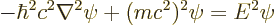This may be rewrit­ten in a form so that both the Schrö­din­ger equa­tion and the Klein-Gor­don equa­tion are cov­ered: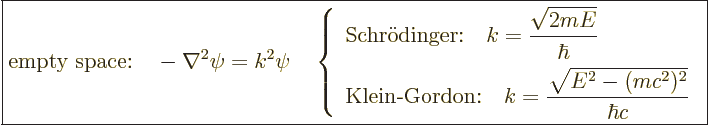(A.44)

Here the con­stant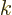is called the wave num­ber. Note that the non­rel­a­tivis­tic en­ergy does not in­clude the rest mass en­ergy. When that is taken into ac­count, the Schrö­din­ger ex­pres­sion forabove is the non­rel­a­tivis­tic ap­prox­i­ma­tion for the Klein-Gor­donas it should be.

Fur­ther note that rel­a­tivis­tic or not, the mag­ni­tude of lin­ear mo­men­tumis given by the “de Broglie re­la­tion”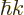. That is be­cause rel­a­tivis­tic or not the mo­men­tum op­er­a­tor is, so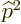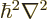. Sim­i­larly, rel­a­tivis­tic or not, the en­ergy is as­so­ci­ated the op­er­a­tor. That means that the time-de­pen­dent fac­tor in states of def­i­nite en­ergy is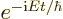. That al­lows the en­ergy to be as­so­ci­ated with an an­gu­lar fre­quency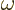by writ­ing the ex­po­nen­tial as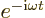. The re­la­tion­ship be­tween en­ergy and fre­quency is then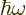. That is known as the “Planck-Ein­stein re­la­tion” when ap­plied to pho­tons. In short, rel­a­tivis­tic or not,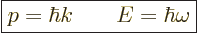(A.45)

The wave num­beris the quan­tum num­ber of lin­ear mo­men­tum, and the an­gu­lar fre­quencyis the one of en­ergy. See ad­den­dum {A.19} for more on how these num­bers arise phys­i­cally from sym­me­tries of na­ture.

It may be noted that Schrö­din­ger wrote down the Klein-Gor­don equa­tion first. But when he added the Coulomb po­ten­tial, he was not able to get the en­ergy lev­els of the hy­dro­gen atom. To fix that prob­lem, he wrote down the sim­pler non­rel­a­tivis­tic equa­tion that bears his name. The prob­lem in the rel­a­tivis­tic case is that af­ter you add the Coulomb po­ten­tial to the en­ergy, you can no longer square away the square root. Even­tu­ally, Dirac fig­ured out how to get around that prob­lem, chap­ter 12.12 and {D.81}. In brief, he as­sumed that the wave func­tion for the elec­tron is not a scalar, but a four-di­men­sion­al vec­tor, (two spin states for the elec­tron, plus two spin states for the as­so­ci­ated anti­elec­tron, or positron.) Then he as­sumed that the square root takes a sim­ple form for that vec­tor.

Since this ad­den­dum as­sumes a par­ti­cle in empty space, the prob­lem with the Coulomb po­ten­tial does not arise. But there are other is­sues. The good news is that ac­cord­ing to the Klein-Gor­don equa­tion, ef­fects do not prop­a­gate at speeds faster than the speed of light. That is known from the the­ory of par­tial dif­fer­en­tial equa­tions. In clas­si­cal physics, ef­fects can­not prop­a­gate faster than the speed of light, so it is some­what re­as­sur­ing that the Klein-Gor­don equa­tion re­spects that.

Also, all in­er­tial ob­servers agree about the Klein-Gor­don equa­tion, re­gard­less of the mo­tion of the ob­server. That is be­cause all in­er­tial ob­servers agree about the rest massof a par­ti­cle and the value of the speed of light. So they all agree about the right hand side in the Klein-Gor­don equa­tion (A.43). And the left hand side in the Klein-Gor­don equa­tion is also the same for all in­er­tial ob­servers. You can crunch that out us­ing the Lorentz trans­form as given in chap­ter 1.2.1 (1.6). (Those fa­mil­iar with in­dex no­ta­tion as briefly de­scribed in chap­ter 1.2.5 rec­og­nize the en­tire left hand side as be­ing sim­ply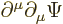. That is un­changed go­ing from one ob­server to the next, be­cause the up­per in­dex trans­forms un­der the Lorentz trans­form and the lower in­dex un­der the in­verse Lorentz trans­form. The op­er­a­tor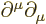is called the “D’Alem­bert­ian,” much like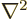is called the Lapla­cian.)

But the bad news is that the Klein-Gor­don equa­tion does not nec­es­sar­ily pre­serve the in­te­gral of the square mag­ni­tude of the wave func­tion. The Schrö­din­ger equa­tion im­plies that, {D.32},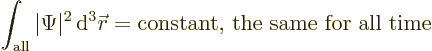The wave func­tion is then nor­mal­ized so that the con­stant is 1. Ac­cord­ing to the Born sta­tis­ti­cal in­ter­pre­ta­tion, chap­ter 3.1, the in­te­gral above rep­re­sents the prob­a­bil­ity of find­ing the par­ti­cle if you look at all pos­si­ble po­si­tions. That must be 1 at what­ever time you look; the par­ti­cle must be some­where. Be­cause the Schrö­din­ger equa­tion en­sures that the in­te­gral above stays 1, it en­sures that the par­ti­cle can­not just dis­ap­pear, and that no sec­ond par­ti­cle can show up out of nowhere.

But the Klein-Gor­don equa­tion does not pre­serve the in­te­gral above. There­fore the num­ber of par­ti­cles is not nec­es­sar­ily pre­served. That is not as bad as it looks, any­way, be­cause in rel­a­tiv­ity the mass-en­ergy equiv­a­lence al­lows par­ti­cles to be cre­ated or de­stroyed, chap­ter 1.1.2. But cer­tainly, the in­ter­pre­ta­tion of the wave func­tion is not a pri­ori ob­vi­ous. The in­te­gral that the Klein-Gor­don equa­tion does pre­serve is, {D.32},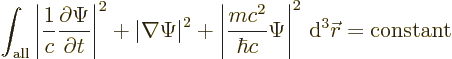It is maybe some­what com­fort­ing that ac­cord­ing to this ex­pres­sion, the in­te­gral of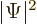must at least re­main bounded. That does as­sume that the rest massof the par­ti­cle is not zero. Pho­tons need not ap­ply.

An­other prob­lem arises be­cause even though the square en­ergy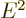is nor­mally pos­i­tive, the en­ergyit­self can still be both pos­i­tive or neg­a­tive. That is a prob­lem, be­cause then there is no lower limit to the en­ergy, there is no ground state. The par­ti­cle can then tran­si­tion to states of lower and lower en­ergy tend­ing to mi­nus in­fin­ity. That would re­lease un­bounded amounts of en­ergy. (Since the ki­netic en­ergy can be ar­bi­trar­ily large, the pos­i­tive value of the en­ergy can be ar­bi­trar­ily large. That makes the neg­a­tive value of the en­ergy also ar­bi­trar­ily large in mag­ni­tude.)

You might say, just ig­nore the neg­a­tive en­ergy pos­si­bil­ity. But Dirac found that that does not work; you need both pos­i­tive and neg­a­tive en­ergy states to ex­plain such things as the hy­dro­gen en­ergy lev­els. The way Dirac solved the prob­lem for elec­trons is to as­sume that all neg­a­tive states are al­ready filled with elec­trons. Un­for­tu­nately, that does not work for bosons, since any num­ber of bosons can go into a state.

The mod­ern view is to con­sider the neg­a­tive en­ergy so­lu­tions to rep­re­sent an­tipar­ti­cles. In that view, an­tipar­ti­cles have pos­i­tive en­ergy, but move back­wards in time. For ex­am­ple, Dirac’s neg­a­tive en­ergy states are not elec­trons with neg­a­tive en­ergy, but positrons with pos­i­tive en­ergy. Positrons are then elec­trons that move back­ward in time. To il­lus­trate the idea, con­sider two hy­po­thet­i­cal wave func­tions of the form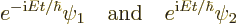whereis the pos­i­tive root for the en­ergy. The first wave func­tion is no prob­lem; it is of the form of a wave func­tion that you would get for a non­rel­a­tivis­tic par­ti­cle of en­ergy. The sec­ond wave func­tion is the prob­lem. It is not con­sid­ered to be a par­ti­cle of neg­a­tive en­ergy. In­stead it is con­sid­ered an an­tipar­ti­cle of pos­i­tive en­ergythat moves back­ward in time. It is the re­ver­sal of the rel­e­vant di­rec­tion of time that causes the sign change in the ar­gu­ment of the ex­po­nen­tial.

You see why so much quan­tum physics is done us­ing non­rel­a­tivis­tic equa­tions.